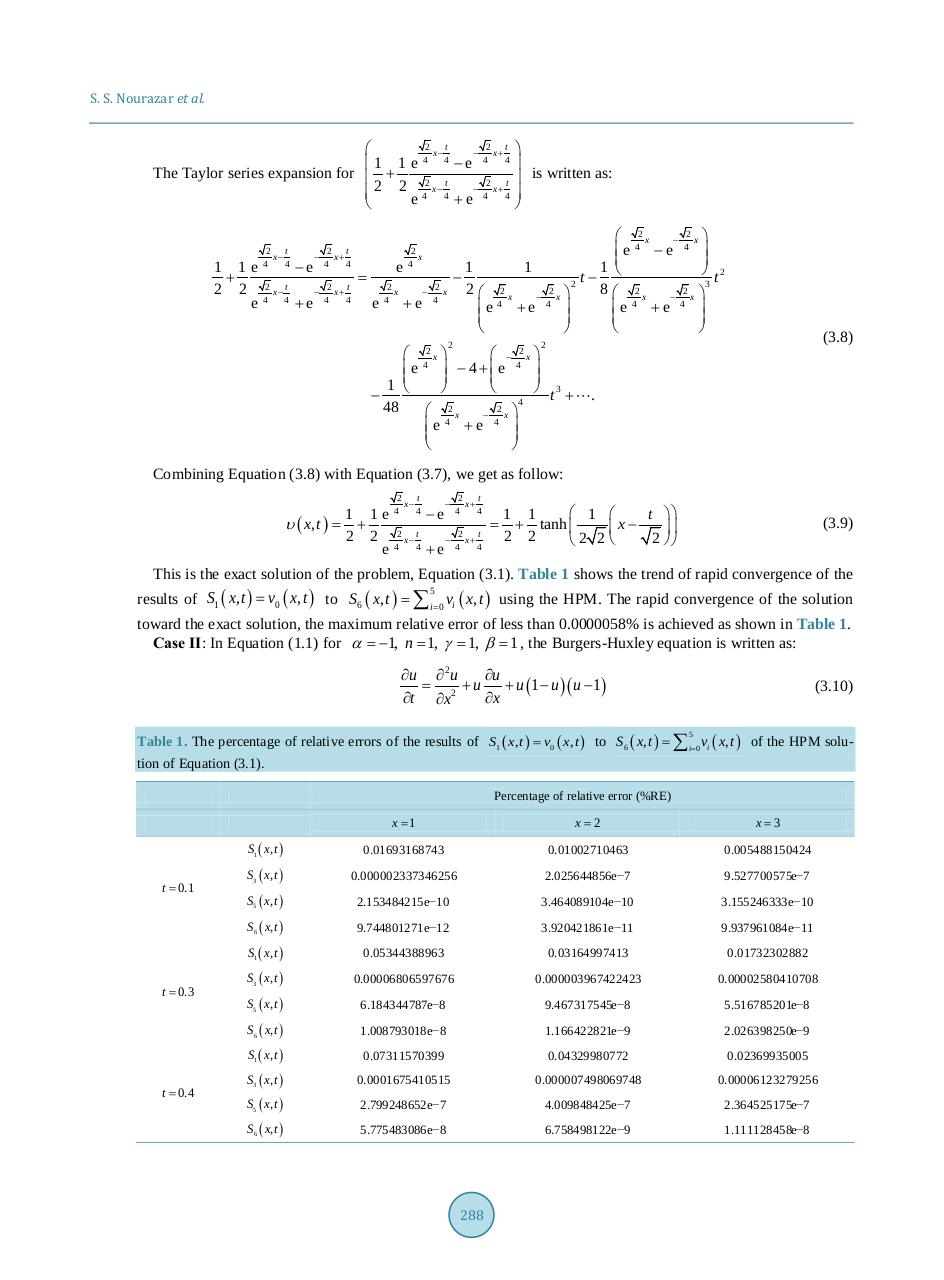# 285 294.pdfPage 1 2 3 4 5 6 7 8 9 10

#### Text preview

S. S. Nourazar et al.

t
2

x−

1 1 e 4 4 −e
The Taylor series expansion for  +
t
2
 2 2 e 4 x − 4 + e−

2
t
x−
4
4

1 1e
−e
+
2
t
2 2
x−

e 4 4 +e

2
t
x+
4
4
2
t
x+
4
4

e
=
2
e

4

x

2
x
4

+e

2
x

4

t
2
x+
4
4
t
2
x+
4
4

1
2
e

2

 is written as:

1
2
x
4

+e

2
x
4

2

2 
 2x

x
e 4 − e 4 

1
 t2
t− 
3
2 
8  2x
x

4
4
+e
e

(3.8)

2

 2x 
 − 2x 
e 4  − 4 +e 4 

1 

 t 3 + .
− 
4
2
2
48

x

x 
e 4 + e 4 

Combining Equation (3.8) with Equation (3.7), we get as follow:
2

x−

t

1 1 e 4 4 −e
υ ( x, t ) =
+
2 2 2 x− t

e 4 4 +e

2
t
x+
4
4
2
t
x+
4
4

 1 
1 1
t 
=
+ tanh 
x−

2 2
2 
2 2

(3.9)

This is the exact solution of the problem, Equation (3.1). Table 1 shows the trend of rapid convergence of the
results of S1 ( x, t ) = v0 ( x, t ) to S6 ( x, t ) = ∑ i = 0 vi ( x, t ) using the HPM. The rapid convergence of the solution
5

toward the exact solution, the maximum relative error of less than 0.0000058% is achieved as shown in Table 1.
Case ІІ: In Equation (1.1) for α =
−1, n =
1, γ =
1, β =
1 , the Burgers-Huxley equation is written as:

∂u ∂ 2 u
∂u
=
+ u + u (1 − u )( u − 1)
∂t ∂x 2
∂x

(3.10)

Table 1. The percentage of relative errors of the results of S1 ( x, t ) = v0 ( x, t ) to S6 ( x, t ) = ∑ i=0 vi ( x, t ) of the HPM solu5

tion of Equation (3.1).
Percentage of relative error (%RE)

t = 0.1

t = 0.3

t = 0.4

x =1

x=2

x=3

S1 ( x, t )

0.01693168743

0.01002710463

0.005488150424

S3 ( x, t )

0.000002337346256

2.025644856e−7

9.527700575e−7

S5 ( x, t )

2.153484215e−10

3.464089104e−10

3.155246333e−10

S 6 ( x, t )

9.744801271e−12

3.920421861e−11

9.937961084e−11

S1 ( x, t )

0.05344388963

0.03164997413

0.01732302882

S3 ( x, t )

0.00006806597676

0.000003967422423

0.00002580410708

S5 ( x, t )

6.184344787e−8

9.467317545e−8

5.516785201e−8

S 6 ( x, t )

1.008793018e−8

1.166422821e−9

2.026398250e−9

S1 ( x, t )

0.07311570399

0.04329980772

0.02369935005

S3 ( x, t )

0.0001675410515

0.000007498069748

0.00006123279256

S5 ( x, t )

2.799248652e−7

4.009848425e−7

2.364525175e−7

S 6 ( x, t )

5.775483086e−8

6.758498122e−9

1.111128458e−8

288